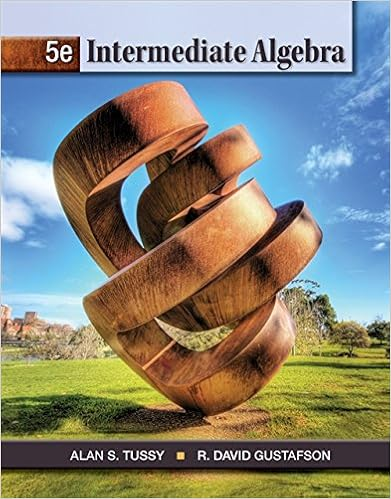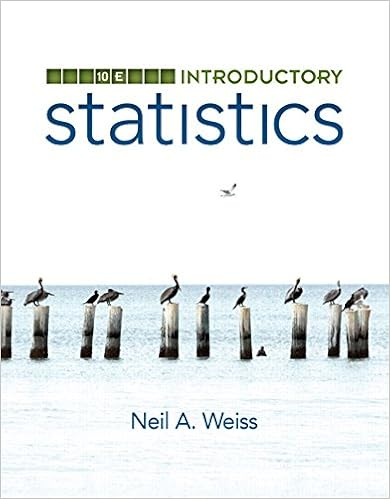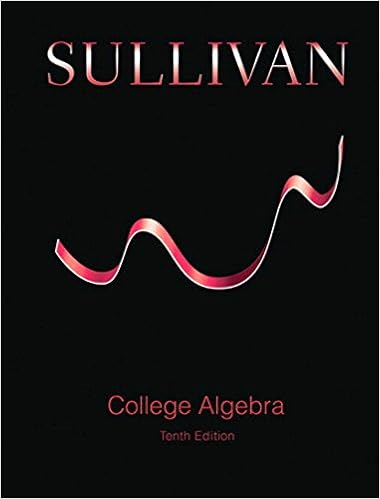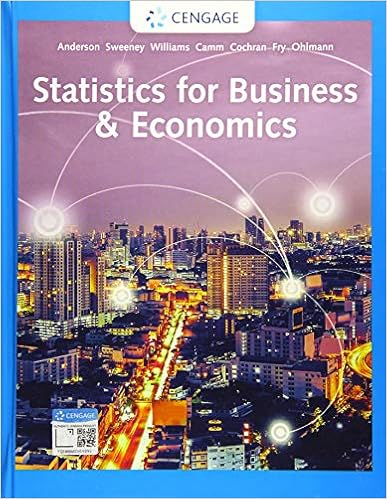# HW12 - MAP 103: Prociency Algebra Homework #12 DUE:...

• Notes
• 1

This preview shows page 1 out of 1 page.

MAP 103: Proficiency AlgebraHomework #12DUE: TUESDAY, NOV. 24, 20091.Letf(x) =x+ 1 andg(x) =12x+ 2.Perform the given operations onf(x) andg(x),specifiedbelow.(a)f(x) +g(x)(b)f(x)-g(x)(c)f(x)·g(x)(d) (*)f(2x) +g(x-1)2.Find a formula for the inverse function to each of the functions given below.(a)f(x) = 2x-3(b)g(x) =12x+ 2(c)m(x) = (x2+ 1)2+ 1(d)n(x) =x-1x+13.Letf(x) =x2+ 1.(a) Find a formula for the inverse off(x).(b) On what domain would the graph off-1(x) be a function? (i.e. pass the vertical line test)4.Consider a relationR={(0,1),(1,2),(2,4),(3,8),(4,16)}.Does the relationRhave an inverse? Ifso, does that meanRalso has an inverse function?5.Letf(x) =1x+1andg(x) =2+xx+3.Find an algebraic expression forf-1(x) +g-1(x).What is thedomain of this new expression?6.Letg(x) =13x+23.Find a formula forg-1(x),the inverse ofg(x),and solve the equationg-1(x) = 2.7.(*) In class, we discussed that it is also possible to get the inverse of a function if we reflected theoriginal graph over the liney=x.In other words, if (x, y) is a point on the original picture, thenwhen it is reflected over the line
##### We have textbook solutions for you!The document you are viewing contains questions related to this textbook.
Chapter 3 / Exercise 26
Intermediate Algebra
Tussy/GustafsonExpert VerifiedBrowse all Textbook Solutions
End of preview. Want to read the entire page?

Course Hero member to access this document

Term
Fall
Professor
Staff
Tags
Expression, Inverse function, 20 000 feet, Pro ciency Algebra Homework
##### We have textbook solutions for you!
The document you are viewing contains questions related to this textbook.The document you are viewing contains questions related to this textbook.
Chapter 3 / Exercise 26
Intermediate Algebra
Tussy/GustafsonExpert Verified
•••